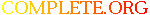Complete.Org: Mailing Lists: Archives: freeciv-dev: February 2003: [Freeciv-Dev] Re: (PR#2370) Path finding# [Freeciv-Dev] Re: (PR#2370) Path finding

[Top] [All Lists]

 To: rf13@xxxxxxxxxxxxxxxxx Subject: [Freeciv-Dev] Re: (PR#2370) Path finding From: "Mike Kaufman" Date: Wed, 26 Feb 2003 21:58:14 -0800 Reply-to: rt@xxxxxxxxxxxxxx

```here are my comments on pf26. Note that I didn't even bother compiling the
code. I'll assume that somebody else is looking over GB's dynamic allocation
implementation to make sure that it ain't broke. I looked at pure API.

Frankly, just using the API reference in path_finding.h, I wouldn't know
where to begin. After some code is actually written using it, a reference
to it needs to be made.

-mike

```
``` *   extra cost (EC): extra cost of a _sinlge_ tile.  EC is always >=
*   0.
single still misspelled.
reformat so 0 is on the previous line.
still not explained. should add "see DISCUSSION below."

*   total_MC: (effective) move cost of the whole path.  Calculated
*   depending on the turn mode (see below).
(effective) has interesting possible connotations none of which are explained
here

*   best path: a path which has the minimal total_CC. If two paths
*   have equal total_CC the behavior is undefined.
not sure I like this. Is it possible to have this? If so define a behavior,
like: the last calculated or the first in the queue or whatever is picked
as the path.

* FORMULAE:
*   For calculating total_MC:
should read: "For calculating total_MC (given the particular tile_behavior):"

*     - TM_NONE: total_MC = sum of MC
lose the "-"

in the EXAMPLES, your (B) above and below are confusing. above, you seem to
know what you want, but don't know where. Below, you say that you don't know
the goal... How is goal used in this context? Clarify.

* The factor which is used to multiple total_EC in the total_CC
* calculation. See definition of total_CC above.
*/
#define PF_TURN_FACTOR  65536

still stands.

int (*get_MC) (int from_x, int from_y, enum direction8 dir,
int to_x, int to_y, struct pf_parameter * param);
comment should reference pf_tools.c for examples.

/* The map itself.  Opaque type. */
struct pf_map;
ah, thank you.

bool pf_get_path(struct pf_map *pf_map, int end_x, int end_y,
struct pf_path *path);
perhaps this should be renamed pf_get_best_path() then?

* Iterates the map until it reaches (x, y).  Then fills the info
* about it into pos.  Returns FALSE if position is unreachable.
* Contents of pos in this case is not defined.
*/
bool pf_get_position(struct pf_map *pf_map, int end_x, int end_y,
struct pf_position *pos);
(x,y) or (end_x, end_y)? if the former, where did these guys come from?

* Saves the next nearest position in an internal buffer. This buffer can be
s/in an internal/to an internal/
also perhaps s/Saves/Appends/ if I'm understanding correctly.

the "internal buffer" is one of those things whose use needs to be explained

path_finding.c:

#define INITIAL_PATH_LENGTH 200
#define INITIAL_DANGER_PATH_LENGTH 50
uh, these should really be cut down; at least by half.

utiny_t *status;              /* Array of node stati
* (enum pf_node_status really) */
I'm fairly sure that the plural is statuses. I got confused for a bit.

pf_tools.h

* Below iterator is mostly for use by AI, iterates through all positions
* on the map, reachable by punit, in order of their distance from punit.
* Returns info about these positions via the second field.
*/
#define simple_unit_path_iterator(punit, position) {
ALL positions?!

#define simple_unit_overlap_path_iterator(punit, position) {
ok, after reading the comment, I'm confident that there's a better name for
this, for example: simple_unit_oncoast_path_iterator or
simple_unit_landocean_path_iterator
```